# Team:Aberdeen Scotland/Bifurcation

University of Aberdeen - ayeSwitch - iGEM 2010

iGEM 2010

# Bifurcation Analysis

The literal meaning of bifurcation is, “division into two parts, branches, or aspects” (Answers). In mathematics, this ‘division’ is in reference to when a non-linear equation suddenly changes its behaviour qualitatively when a parameter of the system is varied. The point at which bifurcation occurs is referred to as the ‘bifurcation point’.

### what is bistability?

If a system is bistable it can be stable in two distinct states. There are also stable oscillations, where the state of the system is stable but not at rest.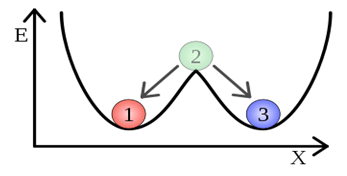Figure 1.This is a graphical representation of bistability. Ball 2 can be equally
'happy' in either the 'one' or 'three' positions. Once it falls into one of these states,
ball 2 will not be inclined to leave this state, hence making it a "stable state".

### Why are they important?

Analyzing the stability of a system is key to understand its behaviour. In biological terms, bistability represents the potential for our system to switch between the expression of green- and cyan-fluorescent proteins. Our goal is to analyze the parameter space in order to determine which parameters are needed to allow for bistability in the system.

A bifurcation diagram has branches which define the possible states a system can be in. By analyzing the bifurcation plots, we can determine how far apart the two “branches”, or stable states, are. The further apart the branches, the harder it will be to attain a switch between the two stable states, however the switch also becomes more stable with increased distance between the branches. Therefore, we want to manipulate the parameters in the system to guarantee robust bistability.

### How do you analyse the stability of a system?

In order to analyze the stability of the system, we utilized XPP Auto, C programming, and MATLAB. We will attempt to detail the math behind what the program does computationally.

The process involved with general stability analysis is as follows. We have taken a simple example system that is easy to analyze in order to demonstrate the general process:

Take the following example system of two differential equations: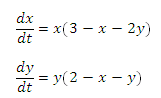#### 1. Set the differential equations to zero: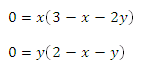#### Find the fixed points of the system of differential equations

There is a fixed point at x = y = 0. By observation, we can see that there are fixed points at x = 0, y = 2, and x = 3, y=0. Finally, by solving this system of equations, we see that there is also a fixed point at x=1 and y=1.

#### 3. Evaluate the Jacobian matrix of the system at these fixed points:

If we label the top function f1 and the bottom f2, the Jacobian of the system will be of the form: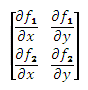When we evaluate the Jacobian matrix for our example system of equations, we get: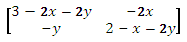#### 4. Using the Jacobian matrix, find the corresponding eigenvalues:

Definition: An eigenvalue is a value λ such that Ax = λx, where x is said to be an eigenvector of A. In order to solve for λ, solve the following equation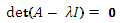where det is the determinant of the matrix and I is the identity matrix. This is known as the characteristic equation of a matrix.

In the case of our example system, the eigenvalues are: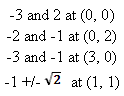#### 5. Take the signs of these eigenvalues in order to analyse stability:

The eigenvalues of a system give important information about the stability of the system. The stability of each of the fixed points can be analyzed based on the following criteria:

 Positive real and complex parts : Unstable Negative real and complex parts : Stable Positive real part : Unstable spiral (spiraling out) Negative real part : Stable spiral (spiraling inwards)

So, for our example system, it can be deduced that the stability at the fixed points is as follows:

One positive and one negative eigenvalue at (0,0) means that (0,0) is a saddle node
Two negative eigenvalues at (0,2) means that (0,2) is a stable node
Two negative eigenvalues at (3,0) means that (3,0) is a stable node
One positive and one negative eigevalue at (1,1) means that (1,1) is a saddle node

### The simulations involved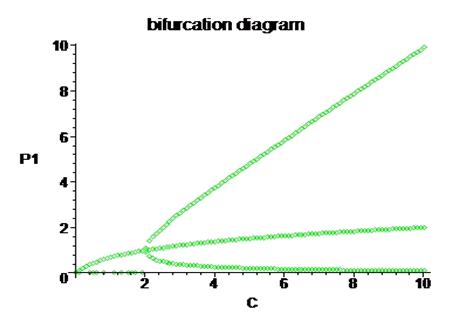Figure 2. Figure 2: This is a bifurcation diagram that we generated using Maple. It ranges over the parameter space of
P1 and C (protein 1, or GFP, and a dimensionless group of parameters) and plots the fixed points of the system at those values.

Where there are three fixed points the system will exhibit bistability. We also know that the further apart the two outer ‘prongs’ the more stable the two definitive fixed states will be, however this makes it more difficult to switch between states.

Back to the Top